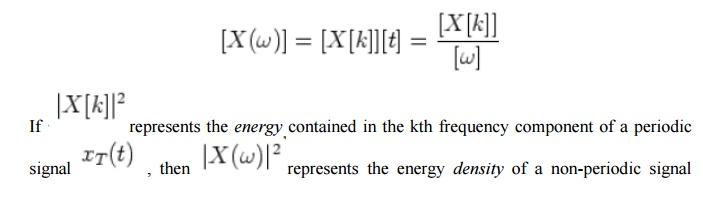Home | | Signals and Systems | Continuous Time Fourier Transform

# Continuous Time Fourier Transform

Fourier expansion coefficient x[k]:

Continuous Time Fourier Transform:

The Fourier expansion coefficient x[k] ( in ak  OWN) of a periodic signal isand the Fourier expansion of the signal is:which can also be written as:When the period of xT(t) approaches infinity, T->(infinity) the periodic signal xT(t) becomes a non-periodic signal and the following will result:

Interval between two neighboring frequency components becomes zero:Discrete frequency becomes continuous frequency:Summation of the Fourier expansion in equation (a) becomes an integral:the second equal sign is due to the general fact:Time integral over in equation (b) becomes over the entire time axis:In summary, when the signal is non-periodic x(t)=limT->infxT(t)  the Fourier expansion becomes Fourier transform. The forward transform (analysis) is:and the inverse transform (synthesis) is:Comparing Fourier coefficient of a periodic signal  xT(t) withwith Fourier spectrum of a non-periodic signal x(t)we see that the dimension of X(ω) is different from that of     X[k]:x(t) distributed along the frequency axis. We can only speak of the energy contained in a particular frequency band ω1< ω< ω2

Study Material, Lecturing Notes, Assignment, Reference, Wiki description explanation, brief detail
Signals and Systems : Classification of Signals and Systems : Continuous Time Fourier Transform |